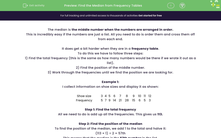# Find the Median from Frequency Tables

In this worksheet, students will practise finding the median from frequency tables and grouped frequency tables.Key stage:  KS 4

Year:  GCSE

GCSE Subjects:   Maths

GCSE Boards:   AQA, Eduqas, Pearson Edexcel, OCR,

Curriculum topic:   Statistics

Curriculum subtopic:   Statistics Analysing Data

Difficulty level:#### Worksheet Overview

The median is the middle number when the numbers are arranged in order.

This is incredibly easy if the numbers are just a list. All you need to do is order them and cross them off from each end.

It does get a bit harder when they are in a frequency table.

To do this we have to follow three steps:

1) Find the total frequency (this is the same as how many numbers would be there if we wrote it out as a list).

2) Find the position of the middle number.

3) Work through the frequencies until we find the position we are looking for.

Example 1:

I collect information on shoe sizes and display it as shown:

 Shoe size 3 4 5 6 7 8 9 10 11 12 Frequency 5 7 9 14 21 28 15 6 5 3

Step 1: Find the total frequency

All we need to do is add up all the frequencies. This gives us 113.

Step 2: Find the position of the median

To find the position of the median, we add 1 to the total and halve it:

(113 + 1) ÷ 2 = 57th

This means that the median is the 57th number in the list.

Step 3: Find the value of the median

To do this, the easiest way is to find the cumulative frequencies then see where the 57th number lies:

 Shoe size 3 4 5 6 7 8 9 10 11 12 Cumulative frequency 5 12 21 35 56 84 99 105 110 113

We can now see that shoe size 7 starts at the 56th number and ends at the 83rd number.

The 57th number must therefore be in the shoe size 7 column.

Example 2:

I collect information on the pocket money of 45 students and display it in a grouped frequency table:

 Pocket money (p) 0 ≤ p < 10 10 ≤ p < 20 20 ≤ p < 30 30 ≤ p < 40 40 ≤ p < 50 50 ≤ p < 60 Frequency 4 6 11 10 8 6

Find the median group and estimate the median pocket money

To do these two tasks we start the same way as we did before:

Step 1: Find the total frequency

All we need to do is add up all the frequencies. This gives us 45.

Step 2: Find the position of the median

To find the position of the median, we add 1 to the total and halve it:

(45 + 1) ÷ 2 = 23

This means that the median is the 23rd number in the list.

Step 3: Find the value of the median

To do this, the easiest way is to find the cumulative frequencies then see where the 23rd number lies:

 Pocket money (p) 0 ≤ p < 10 10 ≤ p < 20 20 ≤ p < 30 30 ≤ p < 40 40 ≤ p < 50 50 ≤ p < 60 Frequency 4 6 11 10 8 6 Cumulative frequency 4 10 21 31 39 45

We can now see that 30 ≤ p < 40 starts at the 22nd number and ends at the 31st number.

The 23rd number must therefore be in the column 30 ≤ p < 40

This is the median group.

Estimating the median (Highest level only)

To estimate the median, we need to know where in this group the 23rd lies.

We know the group starts at the 22nd position and we are looking for the 23rd, so we need to go two positions into this group. (22 and 23)

The group starts at 30 and finishes at 40, so we know it is worth 10.

We can therefore go 2/10 or (1/5th into the group).

As the group is 10 wide, we need to find 1/5 of 10 which is 2.

Adding this onto the start of the group, gives an estimated median of 30 + 2 = £32

Let's try some questions now.

### What is EdPlace?

We're your National Curriculum aligned online education content provider helping each child succeed in English, maths and science from year 1 to GCSE. With an EdPlace account you’ll be able to track and measure progress, helping each child achieve their best. We build confidence and attainment by personalising each child’s learning at a level that suits them.

Get started